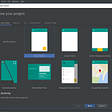# Plus One Problem

Overview

In this particular LeetCode problem, Plus One, I would have to approach it with a crafty approach. The problem states

`You are given a large integer represented as an integer array digits, where each digits[i] is the ith digit of the integer. The digits are ordered from most significant to least significant in left-to-right order. The large integer does not contain any leading 0's.Increment the large integer by one and return the resulting array of digits.`

Here is an example for a better understanding.

`Input: digits = [1,2,3]Output: [1,2,4]Explanation: The array represents the integer 123.Incrementing by one gives 123 + 1 = 124.Thus, the result should be [1,2,4].`

Let's Dive in.

Since we are targeting the end of the array. My first approach was to reverse loop through the array.

`var plusOne = function(digits) {         // reverse loop     for(let i = digits.length - 1; i >= 0; i--){}    };`

Since we can't have double-digit numbers I targeted 9. If the current digit does not equal the value or type of 9 increments by one

`var plusOne = function(digits) {         // reverse loop     for(let i = digits.length - 1; i >= 0; i--){        // add constraints         if(digits[i] !== 9){            digits[i] ++        }            }    };`

Along with incrementing the number, I decided to return the digit.

`var plusOne = function(digits) {         // reverse loop     for(let i = digits.length - 1; i >= 0; i--){        // add constraints         if(digits[i] !== 9){            digits[i] ++            return digits        }            }    };`

I created a SafeNet for 0. If the current number = 0 and if the index is at the current position of 0. Take the array add 1 to it and return the digits.

`var plusOne = function(digits) {         // reverse loop     for(let i = digits.length - 1; i >= 0; i--){        // add constraints         if(digits[i] !== 9){            digits[i] ++            return digits        }        digits[i] = 0        if(i === 0){            digits.unshift(1)            return digits        }    }    };`

--

--

--

## More from Woodelin florveus

Love podcasts or audiobooks? Learn on the go with our new app.

## Keeping Pumps Pumping## We made a stories App and here is a story of how we made it## 2020: Save 20% on Your First Year of GSuite## Session Notes: Re-engineering inside the lines: How to innovate within a legacy stack## Today I Learn About CSS Position Property## 5 Essential Reasons for E2E Testing## Android fundamentals 10.1 Part A: Room, LiveData, and ViewModel## How To Install Docker on Ubuntu 19.10## What Is a Shared Pool In Oracle?## Is pair programming a match for you?## Two bits on `this`